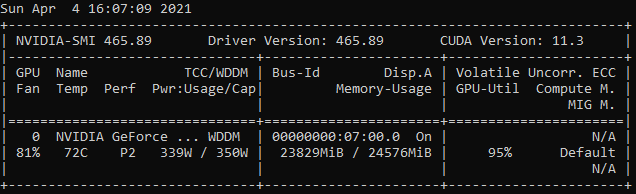# Fine-Tune EleutherAI GPT-Neo to Generate Netflix Movie Descriptions in Only 47 Lines of Code

`pip install git+https://github.com/huggingface/transformers@master`CUDA device used for this project, please note that the GPT-Neo is a very VRAM demanding model!
`# Set the random seed to a fixed value to get reproducible results torch.manual_seed(42)# Download the pre-trained GPT-Neo model's tokenizer# Add the custom tokens denoting the beginning and the end # of the sequence and a special token for paddingtokenizer = GPT2Tokenizer.from_pretrained(“EleutherAI/gpt-neo-1.3B”,                                bos_token=’<|startoftext|>’,                            eos_token=’<|endoftext|>’,                            pad_token=’<|pad|>’)# Download the pre-trained GPT-Neo model and transfer it to the GPUmodel = GPTNeoForCausalLM.from_pretrained("EleutherAI/gpt-neo-1.3B")                         .cuda()# Resize the token embeddings because we've just added 3 new tokens model.resize_token_embeddings(len(tokenizer))`
`descriptions = pd.read_csv(‘netflix_titles.csv’)[‘description’]max_length = max([len(tokenizer.encode(description)) for description in descriptions])`
`class NetflixDataset(Dataset):    def __init__(self, txt_list, tokenizer, max_length):        self.input_ids = []        self.attn_masks = []        self.labels = []        for txt in txt_list:            # Encode the descriptions using the GPT-Neo tokenizer            encodings_dict = tokenizer(‘<|startoftext|>’                                         + txt +                                            ‘<|endoftext|>’,                                        truncation=True,                                        max_length=max_length,                                         padding=”max_length”)        input_ids = torch.tensor(encodings_dict[‘input_ids’])            self.input_ids.append(input_ids)        mask = torch.tensor(encodings_dict[‘attention_mask’])        self.attn_masks.append(mask)def __len__(self): return len(self.input_ids)def __getitem__(self, idx): return self.input_ids[idx], self.attn_masks[idx]`
`dataset = NetflixDataset(descriptions, tokenizer, max_length)`
`train_size = int(0.9 * len(dataset))train_dataset, val_dataset = random_split(dataset,                             [train_size, len(dataset) — train_size])`
`# Here I will pass the output directory where # the model predictions and checkpoints will be stored, # batch sizes for the training and validation steps, # and warmup_steps to gradually increase the learning ratetraining_args = TrainingArguments(output_dir=’./results’,                                  num_train_epochs=5,                                  logging_steps=5000,                                  save_steps=5000,                                                                     per_device_train_batch_size=2,                                  per_device_eval_batch_size=2,                                  warmup_steps=100,                                  weight_decay=0.01,                                    logging_dir=’./logs’)`
`trainer = Trainer(model=model, args=training_args,                    train_dataset=train_dataset,                  eval_dataset=val_dataset,                   # This custom collate function is necessary                   # to built batches of data                  data_collator=lambda data:               {‘input_ids’: torch.stack([f for f in data]),                      ‘attention_mask’: torch.stack([f for f in data]),               ‘labels’: torch.stack([f for f in data])})# Start training process!trainer.train()`
`# Start every description with a special BOS tokengenerated = tokenizer(“<|startoftext|> “,                         return_tensors=”pt”).input_ids.cuda()# Generate 3 movie descriptionssample_outputs = model.generate(generated,                  # Use sampling instead of greedy decoding                  do_sample=True,                  # Keep only top 50 token with                  # the highest probability                 top_k=50,                  # Maximum sequence length                 max_length=300,                  # Keep only the most probable tokens                  # with cumulative probability of 95%                 top_p=0.95,                  # Changes randomness of generated sequences                 temperature=1.9,                 # Number of sequences to generate                                  num_return_sequences=20)# Print generated descriptionsfor i, sample_output in enumerate(sample_outputs):     print(“{}: {}”.format(i, tokenizer.decode(sample_output,                                skip_special_tokens=True)))`